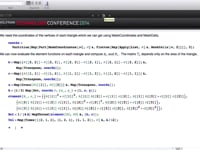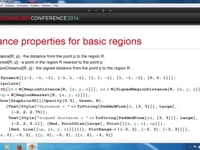Learn how »

# Spectral Zeta Functions

When the eigenvalues of an operator A can be computed and form a discrete set, the spectral zeta function of A reduces to a sum over eigenvalues, when the sum exists. Belloni and Robinett used the quantum bouncer to compute quantum sum rules. In this presentation we use Mathematica to compute the spectral zeta function via the Weierstrass product theorem.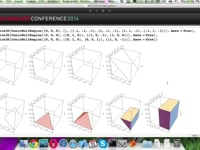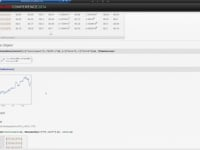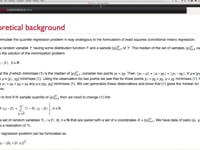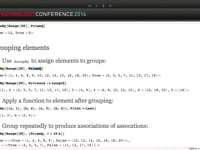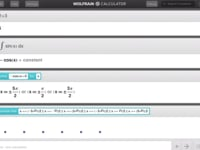iOS23:13Expert Panel53:38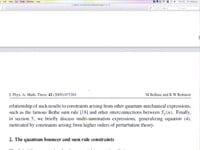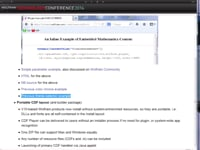What's New in CDF21:27